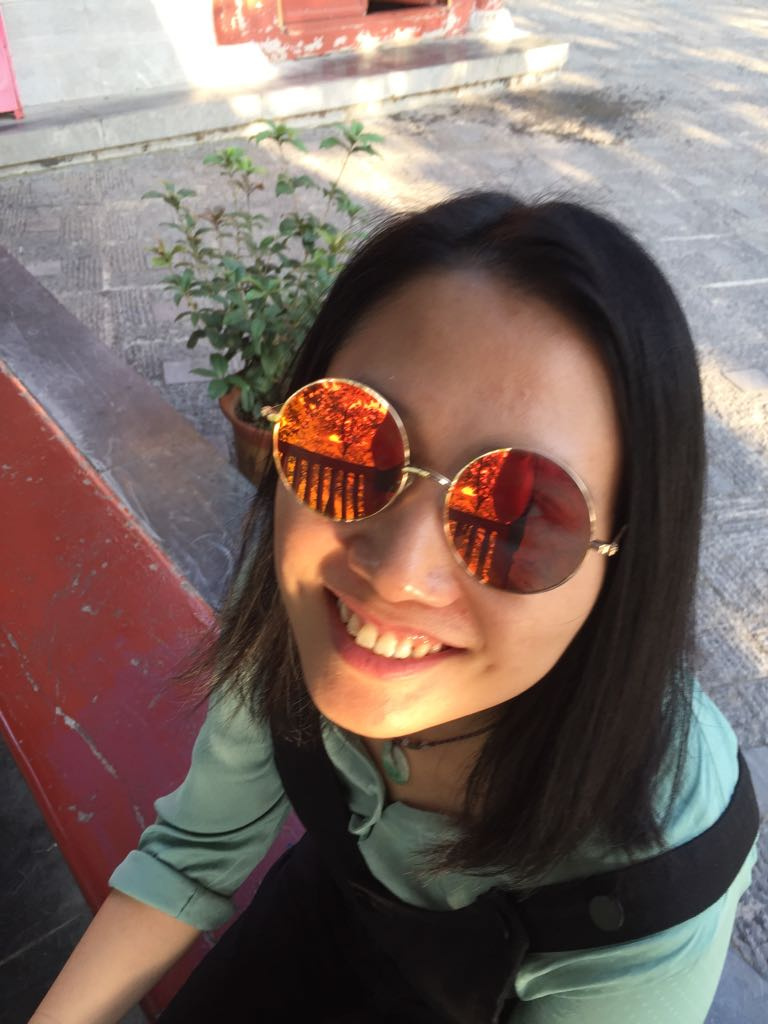# Animated scribbles

Yes! Another animation! I saw the post of Antonio Sánchez Chinchón about his ‘frankenstein scribble’ (Link to his blogpost) and immediatly thought this would like very nice when animated. Antonio uses the Travelling Salesman Problem and the `TSP` package to make a scribble based on a picture. The Basics from Antonio’s code stay the same. So, let’s see how to animate it!

``````library(tidyverse)
library(imager)
library(scales)
library(TSP)
library(gganimate)
library(tweenr)
library(animation)
``````

Instead of Frankenstein, let’s use this picture of my girlfriend this time. Hopefully the end result will look nice enough.

``````file <- "picture.jpg"
``````The pictures is converted to a gray scale picture and then to a matrix of data points we can use for the TSP algorithm.

``````# Load, convert to grayscale
grayscale() %>%
as.matrix

# Convert the matrix to data frame
dimnames(x) = list(row = 1:nrow(x), col = 1:ncol(x))
x <- reshape2::melt(x)
colnames(x)=c("x","y","value")
``````

The next bit of code takes 200 samples of 400 points from the matrix. Each sample contains 400 pairs of x and y coordinates. With the `TSP` package the shortest route between these points is calculated. This route is later used as the order in which the lines are drawn. Instead of adding all these layers to a `ggplot` object, we store the information in a list of data frames. Then all these data frames are combined into one. And we add some extra information that will be used later for animating the data.

``````# start df
data_to_animate <- list()

# This loop adds layers to the plot
for (i in 1:200){

print(paste(i,"out of 200"))
# Weighted sample of pixels
data <- x %>%
sample_n(400, weight=1-value) %>%
select(x,y)

# Compute distances and solve TSP
sol <- as.TSP(dist(data)) %>%
solve_TSP(method = "arbitrary_insertion") %>%
as.integer()

# # Create a dataframe with the output of TSP
order <- data.frame(id = sol)
order <- order %>% mutate(order = 1:nrow(data))

# Rearrange the original points according the TSP output

data2 <- data %>%
mutate(id = 1:nrow(data)) %>%
inner_join(order, by="id") %>% arrange(order)

# specifying attributes for the animation
data_to_animate[[i]] <- data2 %>%
mutate(line = i,
alpha = runif(1, min = 0, max = 0.10)) %>%
select(line, order, x, y, alpha)

}

data_anim <- do.call(bind_rows, data_to_animate)
plot_data <- data_anim %>%
mutate(alpha = as.numeric(alpha),
ease = "linear")
``````

Now the animating begins! The next functions prepares the data for animating. The positions in between the ‘order’ points of the lines is calculated for a smoother animation. And then we calculate a dataframe with a timepoint when the lines should appear in the animation.

``````plot_data_tween <- tween_elements(plot_data, time = "order",  group = "line", ease = "ease", nframes = 200)
df_tween_appear <- tween_appear(plot_data_tween, time = 'order', nframes = 200)
``````

The animation is basicly a loop and makes a plot for each timepoint in the animation. All of the images are then added together. I added a simple progress message as the animating process can take a while…

``````oopt<-ani.options(interval=1/10, ani.width = 200, ani.height = 250)
saveGIF({for (i in 1:max(df_tween_appear\$.frame)){
plot_data <-
df_tween_appear %>% filter(.frame == i, .age > -0)
g <- plot_data %>%
ggplot()+
geom_path(aes(x = x, y = y, group = .group), alpha = 0.08) +
theme_void() +
theme(legend.position="none")+
## to flip the coordinates, otherwise the picture ends up being upside down
scale_y_continuous(trans=reverse_trans())

print(g)
print(paste(i,"out of",max(df_tween_appear\$.frame)))
ani.pause()
}
},movie.name="animition.gif", width = 4, height = 5)
``````And as you can see in the end, the animation works pretty well!

Of course with a bigger resolution and more frames the animation looks smoother and you will see the individual lines scribbled on more clearly. But this can take a looong time. I tried to include the random alpha level for each line. But this didn’t seem to work. I also tried to rewrite the animation in the new `gganimate` syntax, but i couldn’t get it to work properly. The `geom_path` will always connect the first point with the last point directly in combination with the `transition_reveal` command for example. Maybe someone else has an idea how to fix this?

In the end this was a fun animation to work on and with an awesome result!